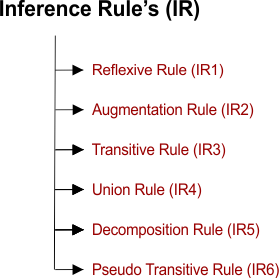Select Page

# Inference Rule (IR)

• Using the inference rule, we can derive additional FD (functional dependency) from the initial attribute set.

## Types Of Inference Rule

The FD (Functional dependency) has 6 types of inference rule. Any rule can be used in-between two attributes### 1. Reflexive Rule (IR1)

• In the reflexive rule, if B is a subset of A, then A determines B.
• Every attribute can determine itself also called Reflexive.
• If A ⊇ B then A  →   B

Example:

A ⊇  A , B  →  B  , AB  →    B

### 2. Augmentation Rule (IR2)

• The augmentation rule is also called as a partial dependency.
• If A    → B then AC   →   BC

### 3. Transitive Rule (IR3)

• In the transitive rule, if A determines B and B determine C, then A must also determine C.
• If A   →   B and B  →  C, then A  →   C

### 4. Union Rule (IR4)

• Union rule says, if A determines B and B determines C, then A must also determine B and C.
• If A  →  B and A   →  C then A  →    BC

### 5. Decomposition Rule (IR5)

• Decomposition rule is also called project rule. It is the reverse of union rule.
• If A → BC then A → B and A →    C

### 6. Pseudo transitive Rule (IR6)

• According to Pseudo transitive Rule
• If A   → B and BC   →   W then AC   →   W
Help Other’s By Sharing…